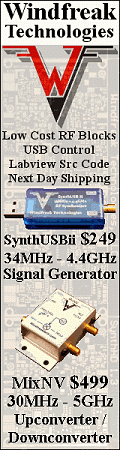Please Support RF Cafe by purchasing my  ridiculously low−priced products, all of which I created.

RF Workbench  (shareware)# Electronic Puzzle SquareJanuary 1945 Radio-Craft

 January 1945 Radio-Craft[Table of Contents] Wax nostalgic about and learn from the history of early electronics. See articles from Radio-Craft, published 1929 - 1953. All copyrights are hereby acknowledged.

This Electronic Puzzle Square, compliments of Lt. C.K. Johnson, appeared in the January 1945 issue of Radio-Craft magazine. It consists of electronics-related numerical questions whose answers, if correct, create a 4x4 matrix whose columns, rows, and long diagonals all add up to 45. Non-integer answers need to be rounded according to standard rules (do not just truncate). In case you left your slide rule at the office, a link is provided to assist with Q2. The biggest head scratcher question is Q4, since it requires the conversion from polar to rectangular coordinates, but hey, you're an engineer (or a crack technician). Having Euclid on-hand for Q5 might be helpful. A link is provided to a copper wire parameter table for Q9. Have fun.

Here is another Electronic Puzzle Square from the July 1945 issue.

Electronic Puzzle SquareBy Lt. C. K. Johnson

Here is a new idea in a Magic Square. Work problem No. 1 and put the answer in square No. 1. The answer to problem 2 goes in square 2, etc. When all 16 problems are completed, the rows, columns diagonals and various other combinations will total a number which is the type number of a popular power amplifier triode frequently used push-pull to give a power output of about 18 watts with 275 volts on the plate.

The numbers add up to 45 in every direction, if answers are correct.

1. The total resistance between A and B is ____ ohms.2. On a slide rule, 64 on the right hand side of the A scale is directly over ____ on the D scale.

3. The pentode is a ____ element tube.

4. 21.18/8.1° would equal ____ +3j in rectangular coordinates.

5. If a regular hexagon is inscribed in a circle whose radius is one inch, the perimeter of the hexagon is ____ inches.

6. What is the voltage between A and B?7. Two 12 volt batteries hooked in parallel will give ____ volts.

8. 0.142857 is the reciprocal of _____.

9. B&S gage bare copper wire No. ____ has a circular mil area of 1,288 and at 25 °C. has a resistance of 8.1 ohms per 1,000 feet. (see table here)

10. To find wavelength in meters when frequency in kilocycles is known, we have a formula λ = (c x 105)/f . What digit does "c" replace?

11. In the R.M.A. color code, silver represents a tolerance of what percent?

12. What is the total capacitance in mfd. between A and B?13. Effective voltage (in alternating current of sine-wave form) is to average voltage as 10 is to ____ .

14. What will be the voltage across the 1-megohm resistor exactly 1.967 seconds after the switch (S1) is closed, allowing current to enter the resistor-condenser combination?15. In this circuit the problem is to find the current in milliamperes if 1.62 watts is dissipated in the 500-ohm resistor.16. Yellow is ____ in the color code.

Posted  February 25, 2021